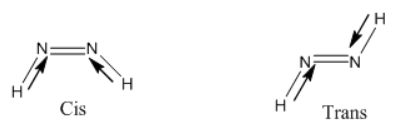×
Get Full Access to Introductory Chemistry - 5 Edition - Chapter 10 - Problem 92p
Get Full Access to Introductory Chemistry - 5 Edition - Chapter 10 - Problem 92p

×

# Classify each molecule as polar or nonpolar.(a) N2H2(b) H2O2(c) CF4(d) NO2ISBN: 9780321910295 34

## Solution for problem 92P Chapter 10

Introductory Chemistry | 5th Edition

• Textbook Solutions
• 2901 Step-by-step solutions solved by professors and subject experts
• Get 24/7 help from StudySoup virtual teaching assistantsIntroductory Chemistry | 5th Edition

4 5 1 335 Reviews
28
4
Problem 92P

Classify each molecule as polar or nonpolar.

(a) $$\mathrm{N}_{2} \mathrm{H}_{2}$$

(b) $$\mathrm{H}_{2} \mathrm{O}_{2}$$

(c) $$\mathrm{CF}_{4}$$

(d) $$\mathrm{NO}_{2}$$

Step-by-Step Solution:

Step 1 of 4

a)

Polar compounds:

The electronegative difference between the species in the range of 0.3 to 1.4 is considered polar compounds.

Example: $$\mathrm{HCl}$$ and $$\mathrm{HF}$$

Nonpolar compounds:

The electronegative differences between the species in the range of 0 to 0.2 are considered as non-polar compounds.

The given molecule $$\mathrm{N}_{2} \mathrm{H}_{2}$$.

The electronegativity of nitrogen - 3.0

The electronegativity of hydrogen - 2.1

Total difference - 0.9

The shape of molecules with bond dipoles $$\mathrm{N}_{2} \mathrm{H}_{2}$$ is as follows.Shape of the molecule is nonlinear.

In the transform of the molecule, the net dipole moment is zero therefore, the given molecule is non - polar in the transform.

The net dipole moment is non - zero in cis form.

Therefore, the given molecule is polar in the cis form.

Step 2 of 4

Step 3 of 4

##### ISBN: 9780321910295

Introductory Chemistry was written by and is associated to the ISBN: 9780321910295. This textbook survival guide was created for the textbook: Introductory Chemistry, edition: 5. This full solution covers the following key subjects: classify, molecule, nonpolar, polar. This expansive textbook survival guide covers 19 chapters, and 2046 solutions. Since the solution to 92P from 10 chapter was answered, more than 2479 students have viewed the full step-by-step answer. The full step-by-step solution to problem: 92P from chapter: 10 was answered by , our top Chemistry solution expert on 05/06/17, 06:45PM. The answer to “?Classify each molecule as polar or nonpolar.(a) $$\mathrm{N}_{2} \mathrm{H}_{2}$$(b) $$\mathrm{H}_{2} \mathrm{O}_{2}$$(c) $$\mathrm{CF}_{4}$$(d) $$\mathrm{NO}_{2}$$” is broken down into a number of easy to follow steps, and 13 words.

Unlock Textbook Solution